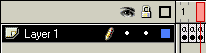# Flash Script – Scrolling Image Gallery

1. Create a blank movie clip, and give it an instance name of "drag".

2. I’ve used a square movieclip and simply made 5 instances of the same clip. For the sake of file size, I’ve used a plain movie clip, but of course, you can use images instead.

Convert each image into a movie clip, and give each instance a name, such as "a1", "a2", "a3", "a4", and "a5".

3. Go to the time line of the "drag" movie clip, and create two key frames.

4. In the first key frame, insert the action:

``````mw = getProperty("/a1",_width);
for(i=5; i>=1; i--)
{

//right side
if(mx > 400)
{
if(i==5){mv=1};
if(i==4){mv=5};
if(i==3){mv=4};
if(i==2){mv=3};
if(i==1){mv=2};
setProperty("/a" add i,_x,mx - (mw + 2));
}

//left side
if(mx < 0)
{
if(i==1){mv=5};
if(i==2){mv=1};
if(i==3){mv=2};
if(i==4){mv=3};
if(i==5){mv=4};
setProperty("/a" add i,_x,mx + (mw + 2));
}
}```

5. In the second key frame, insert the action:

`gotoAndPlay (1); `
6. Go to the main timeline, create a new layer, and name it "actions".
7. Create three keyframes in the "actions" layer.8. In the first key frame, insert the action:
```mx = getProperty("/a1",_x);
mw = getProperty("/a1",_width);
nomc = 5; ```
`9.` Insert the following action into the second key frame:

```mc = _root.drag._x
if (mc>0 and mc<180)
{
for (i=1; i<=nomc; i++)
{
mx = getProperty("/a" add i, _x);
setProperty ("/a" add i, _x, mx+10);
}
}
if (mc>220 and mc<400)
{
for (i=1; i<=nomc; i++)
{
mx = getProperty("/a" add i, _x);
setProperty ("/a" add i, _x, mx-10);
}
}```

10. In the third key frame, insert:

`gotoAndPlay (2); `
12. You're done! Press ctrl+enter to test the movie.
```